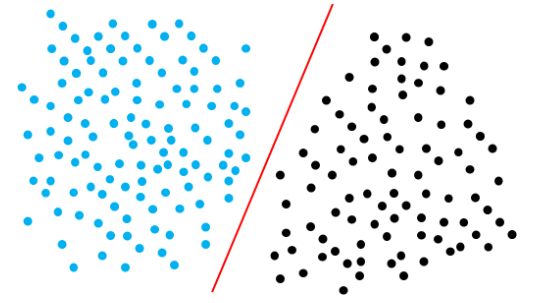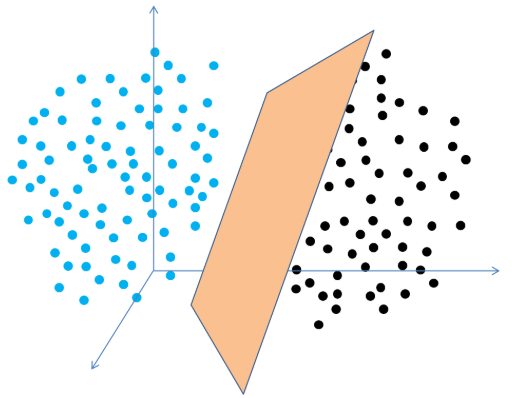# 0x00 线性单元# 0x01 线性模型的表示

$x=\left[ \begin{matrix} 200\\ 100\\ 50 \end{matrix} \right]$

$W=\left[ \begin{matrix} 1&0.5&1.5 \end{matrix} \right]$

$h(x)=Wx=\left[ \begin{matrix} 1&0.5&1.5 \end{matrix} \right]\left[ \begin{matrix} 200\\100\\50 \end{matrix} \right] =1\times200+0.5\times100+1.5\times50$

# 0x02 梯度下降

$e^{(i)}=\frac{1}{2}(y^{(i)}-\bar{y}^{(i)})^2$

$E=\frac{1}{2}\sum_{i=1}^{n}(y^{(i)}-wx^{(i)})^2$

$x_{\text{new}}=x_{\text{old}}-\eta\nabla{f(x)}$

$\mathrm{w}_{\text{new}}=\mathrm{w}_{\text{old}}-\eta\nabla{E(\mathrm{w})}$

$\nabla{E(\mathrm{w})}=\frac{\partial E(\mathrm{w})}{\partial\mathrm{w}}$

$\nabla{E(\mathrm{w})}=-\sum_{i=1}^{n}(y^{(i)}-\bar{y}^{(i)})x^{(i)}$

$\mathrm{w}_{\text{new}}=\mathrm{w}_{\text{old}}+\eta\sum_{i=1}^{n}(y^{(i)}-\bar{y}^{(i)})x^{(i)}$

# 0x03 手写线性拟合

import numpy as np​​class LinearFittingPredictor:    def __init__(self, learn_rate):        self.w = 0        self.b = 0        self.learn_rate = learn_rate​    def get_prediction(self, input_x):        return self.w * input_x + self.b​    def train(self, train_x, train_y, iter):        for _ in range(iter):            self.w = self._train_w(train_x, train_y, self.w)            self.b = self._train_b(train_x, train_y, self.b)​    # 求解E对w的梯度值    def _nabla_E_w(self, train_x, train_y):        sum = 0        for i in range(0, len(train_x)):            sum = sum + (train_y[i] - self.get_prediction(train_x[i])) * train_x[i]        return -sum​    def _nabla_E_b(self, train_x, train_y):        sum = 0        for i in range(0, len(train_x)):            sum = sum + (train_y[i] - self.get_prediction(train_x[i])) * 1        return -sum​    def _train_w(self, train_x, train_y, w_old):        return w_old - self.learn_rate * self._nabla_E_w(train_x, train_y)​    def _train_b(self, train_x, train_y, b_old):        return b_old - self.learn_rate * self._nabla_E_b(train_x, train_y)​​x = np.random.rand(1000, 1)y = 10.21 * x + 12.11​predictor = LinearFittingPredictor(0.001)predictor.train(x, y, 100)​print(predictor.w)print(predictor.b)

[10.20978574][12.11010666]

import numpy as np​​class LinearFittingPredictor:    def __init__(self, learn_rate):        self.w = np.array([0, 0])        self.learn_rate = learn_rate​    def get_prediction(self, input_x):        # 将b看做一个输入x恒为1的特殊的w项        input_x = np.append(input_x, 1).reshape(2, 1)        return self.w.dot(input_x)​    def train(self, train_x, train_y, iter):        for _ in range(iter):            self._train_w(train_x, train_y)​    def _nabla_E_w(self, train_x, train_y):        sum = 0        for i in range(0, len(train_x)):            # 将b看做一个输入x恒为1的特殊的w项            x_i = np.array([train_x[i], 1])            sum = sum + (train_y[i] - self.get_prediction(train_x[i])) * x_i        return -sum​    def _train_w(self, train_x, train_y):        self.w = self.w - self.learn_rate * self._nabla_E_w(train_x, train_y)​​x = np.random.rand(1000, 1)y = 10.21 * x + 12.11​predictor = LinearFittingPredictor(0.001)predictor.train(x, y, 120)​print(predictor.w)

[10.20911554 12.11048001]

# 0x04 下面的你可以当没看见

$\bar{y}=\tanh(wx+b)$

$\mathrm{w}_{\text{new}}=\mathrm{w}_{\text{old}}-\eta\nabla{E(\mathrm{w})}$

$\tanh'(x)=1-\tanh^2(x)$